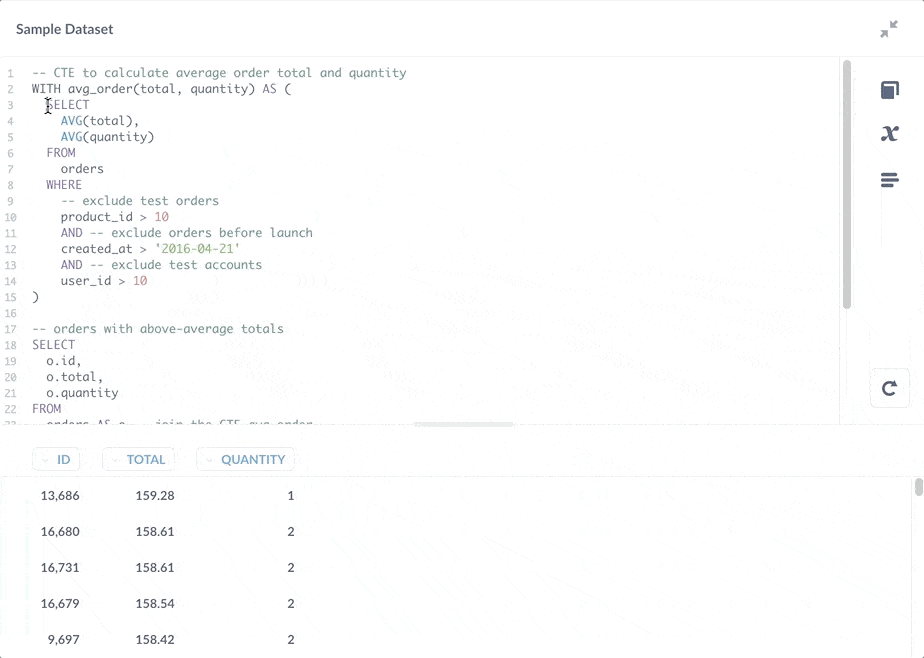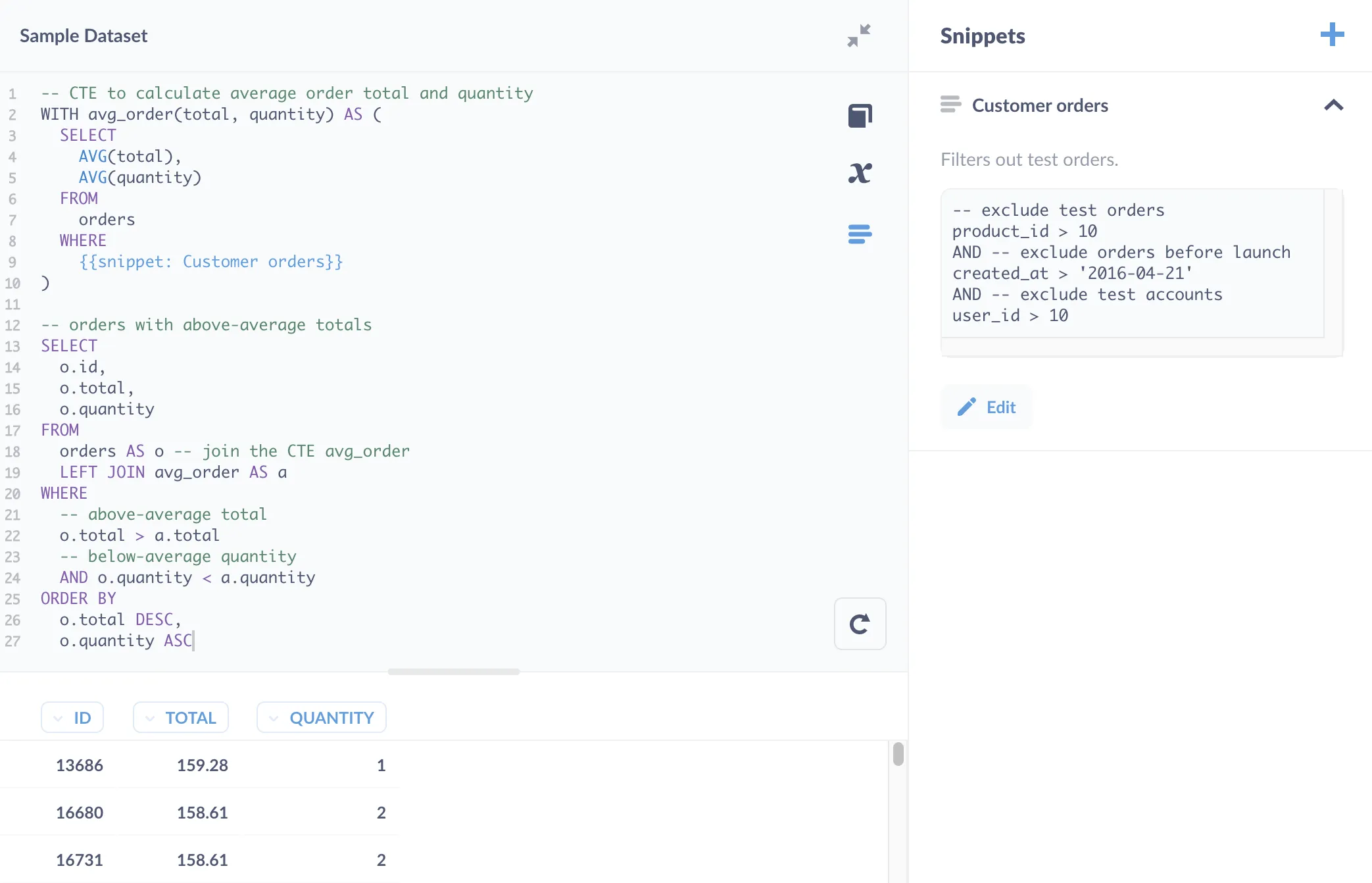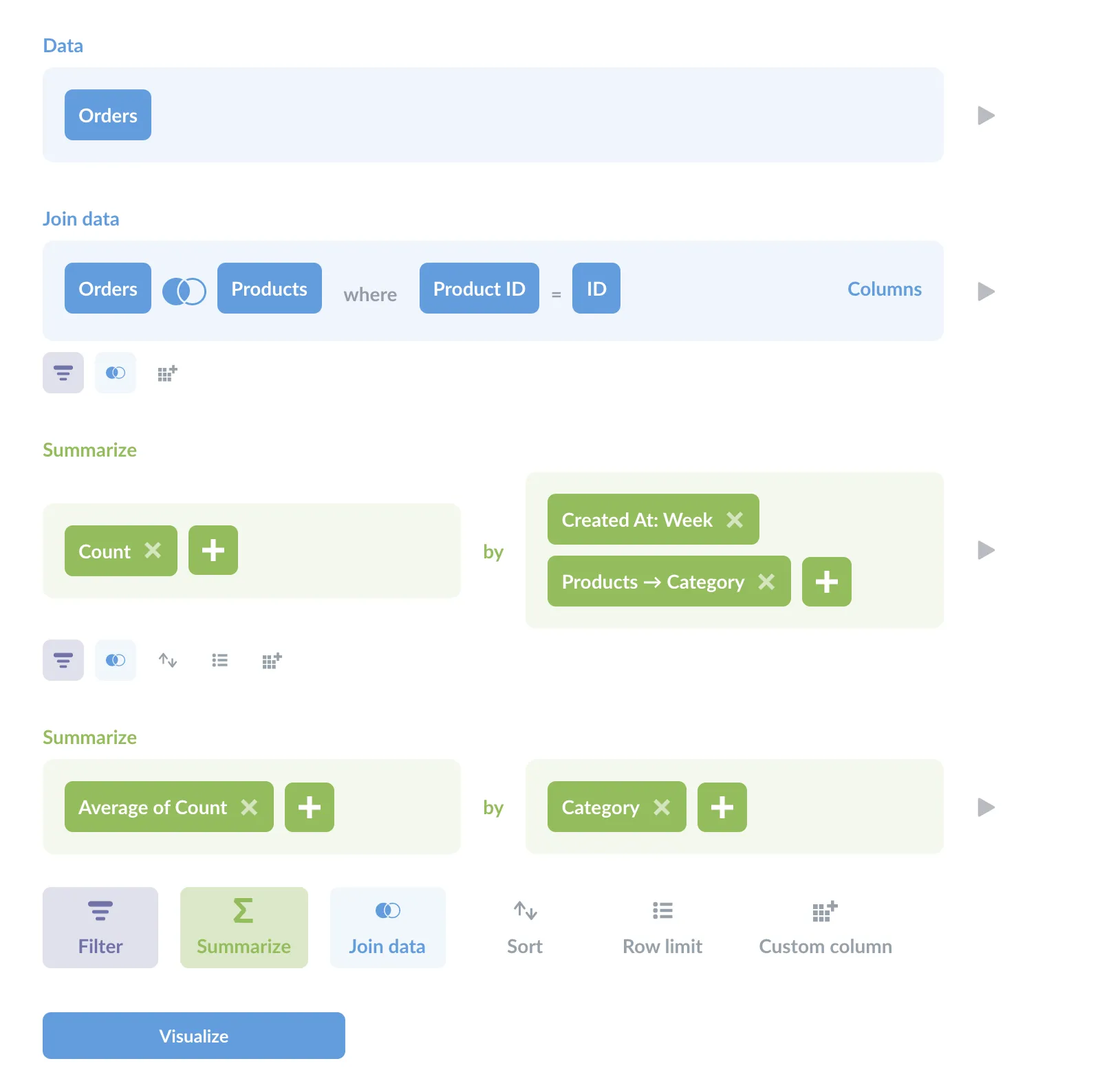# Simplify complex queries with Common Table Expressions (CTEs)

### CTEs are named sets of results that help keep your code organized. They allow you to reuse results in the same query, and perform multi-level aggregations.

A Common Table Expression (CTE) is a named result set in a SQL query. CTEs help keep your code organized, and allow you to perform multi-level aggregations on your data, like finding the average of a set of counts. We’ll walk through some examples to show you how CTEs work and why you would use them, using the Sample Database included with Metabase so you can follow along.

## CTE benefits

• CTEs make code more readable. And readability makes queries easier to debug.
• CTEs can reference the results multiple times throughout the query. By storing the results of the subquery, you can reuse them throughout a larger query.
• CTEs can help you perform multi-level aggregations. Use CTEs to store the results of aggregations, which you can then summarize in the main query.

## CTE syntax

The syntax for a CTE uses the `WITH` keyword and a variable name to create a kind of temporary table that you can reference in other parts of your query.

``````WITH cte_name(column1, column2, etc.) AS (SELECT ...)
``````

The `AS` keyword is a bit unusual here. Normally `AS` is used to specify an alias, like `consumables_orders AS orders`, with `orders` being the alias to the right of `AS`. With CTEs, the variable `cte_name` precedes (is on the left side of) the `AS` keyword, followed by the subquery. Note that the column list `(column1, column2, etc)` is optional, provided that each column in the `SELECT` statement has a unique name.

## CTE example

Let’s go through a simple example. We’d like to see a list of all orders with a `total` that’s greater than the average order’s total.

``````SELECT
id,
total
FROM
orders
WHERE
-- filter for orders with above-average totals
total > (
SELECT
AVG(total)
FROM
orders
)
``````

This query gives us:

``````|ID  |TOTAL  |
|----|-------|
|2   |117.03 |
|4   |115.22 |
|5   |134.91 |
|... |...    |
``````

It seems simple enough: we have a subquery, `SELECT AVG(total) FROM orders`, nested in the `WHERE` clause that calculates the average order total. But what if grabbing the average were more involved? For example, suppose you need to filter out test orders, or exclude orders before your application launch:

``````SELECT
id,
total
FROM
orders
WHERE
total > (
-- calculate average order total
SELECT
AVG(total)
FROM
orders
WHERE
-- exclude test orders
product_id > 10
AND -- exclude orders before launch
created_at > '2016-04-21'
AND -- exclude test accounts
user_id > 10
)
ORDER BY
total DESC
``````

Now the query starts losing legibility. We can rewrite the subquery as a common table expression using a `WITH` statement to encapsulate the results from that subquery:

``````-- CTE to calculate average order total
-- with the name for the CTE (avg_order) and column (total)
WITH avg_order(total) AS (

-- CTE query
SELECT
AVG(total)
FROM
orders
WHERE
-- exclude test orders
product_id > 10
AND -- exclude orders before launch
created_at > '2016-04-21'
AND -- exclude test accounts
user_id > 10
)

-- our main query:
-- orders with above-average totals
SELECT
o.id,
o.total
FROM
orders AS o
-- join the CTE: avg_order
LEFT JOIN avg_order AS a
WHERE
-- total is above average
o.total > a.total
ORDER BY
o.total DESC
``````

The CTE packages up the logic for finding the average, and separates that logic from the core query: finding the order IDs with above-average totals. Note the results of this CTE are not saved anywhere; its subquery is executed each time you run the query.

Storing this query as a CTE also makes the query easier to modify. Let’s say that we also wanted to know which orders have:

• above-average totals,
• below-average quantities of items ordered.

We can easily expand the query like so:

``````-- CTE to calculate average order total and quantity
WITH avg_order(total, quantity) AS (
SELECT
AVG(total),
AVG(quantity)
FROM
orders
WHERE
-- exclude test orders
product_id > 10
AND -- exclude orders before launch
created_at > '2016-04-21'
AND -- exclude test accounts
user_id > 10
)

-- orders with above-average totals
SELECT
o.id,
o.total,
o.quantity
FROM
orders AS o -- join the CTE avg_order
LEFT JOIN avg_order AS a
WHERE
-- above-average total
o.total > a.total
-- below-average quantity
AND o.quantity < a.quantity
ORDER BY
o.total DESC,
o.quantity ASC
``````

We can also select and run only the subquery in the CTE.Fig. 1. Highlighting part of a query in a CTE and running the selection to see its results.

As you can see in the figure above, you can also save the CTE’s subquery as a snippet, but you’d be better off saving a subquery as a question. The rule of thumb to decide between a snippet and a saved question is that if a block of code can return results on its own, you may want to consider saving it as a question (see SQL snippets vs saved questions vs views).

A better case for a SQL snippet would be the `WHERE` clause that captures the logic for filtering for customer orders.Fig. 2. Inserting a snippet, Customer orders, that filters out test orders and accounts.

## CTE with a saved question

You can use the `WITH` statement to reference a saved question:

``````WITH avg_order(total, quantity) AS {{#2}}

-- orders with above-average totals
SELECT
o.id,
o.total,
o.quantity
FROM
orders AS o -- join the CTE avg_order
LEFT JOIN avg_order AS a
WHERE
-- above-average totals
o.total > a.total
-- below-average quantity
AND o.quantity < a.quantity
ORDER BY
o.total DESC,
o.quantity ASC
``````

You can see the question referenced by the variable `{{#2}}` using the Variables sidebar. In this case, `2` is the question’s ID.

By saving that subquery as a standalone question, multiple questions would be able to reference its results. And if you need to add additional `WHERE` clauses to exclude more test orders from the calculation, each question that references that calculation would benefit from the update. The flipside of this benefit is that if you end up changing that saved question to return different columns, it would break queries that depend on its results.

## CTEs for multi-level aggregations

You can use CTEs to perform multi-level, or multi-stage, aggregations. That is, you can perform aggregations on aggregations, like taking the average of a count.

### Example: what are the average numbers of orders placed each week in each product category?

To answer this question in this section’s title, we’ll need to:

1. Find the count of orders per week in each product category.
2. Find the average count for each category.

You can use a CTE to find the count, then use the main query to calculate the average.

``````-- CTE to find orders per week by product category
WITH orders_per_week(
order_week, order_count, category
) AS (
SELECT
DATE_TRUNC('week', o.created_at) as order_week,
COUNT(*) as order_count,
category
FROM
orders AS o
left join products AS p ON o.product_id = p.id
GROUP BY
order_week,
p.category
)

-- Main query to calculate average order count per week
SELECT
category AS "Category",
AVG(order_count) AS "Average orders per week"
FROM
orders_per_week
GROUP BY
category
``````

Which yields:

``````|Category |Average orders per week|
|---------|-----------------------|
|Doohickey|19                     |
|Gizmo    |23                     |
|Widget   |25                     |
``````

### Multi-level aggregation in the Notebook Editor

Just to provide a birds-eye view of what’s going on in this query, here’s what the above query would look like in Metabase’s Notebook Editor:Fig. 4. Using the Notebook Editor to find the average weekly count of orders by product category. Note the two summarization steps in green.

You can clearly see the two stages of aggregation (the two Summarize sections). As this shows, even when you’re writing SQL queries, the Notebook Editor can be a great tool for poking around the data and helping you plan your approach.

## Using multiple CTEs in a single query

You can use multiple CTEs in the same query. All you need to do is separate their names and subqueries with a comma, like so:

``````-- first CTE
WITH avg_order(total) AS (
SELECT
AVG(total)
FROM
orders
),
-- second CTE (note the preceding comma)
avg_product(rating) AS (
SELECT
AVG(rating)
FROM
products
)
``````